moveit2 The MoveIt Motion Planning Framework for ROS 2.
ompl_interface::BaseConstraint Class Referenceabstract

Abstract base class for different types of constraints, implementations of ompl::base::Constraint. More...

`#include <ompl_constraints.h>`

Inheritance diagram for ompl_interface::BaseConstraint: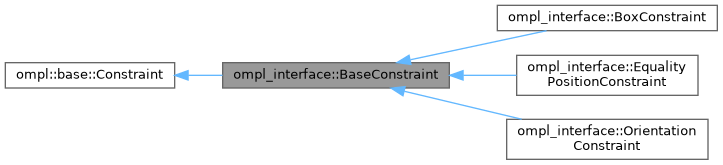[legend]
Collaboration diagram for ompl_interface::BaseConstraint: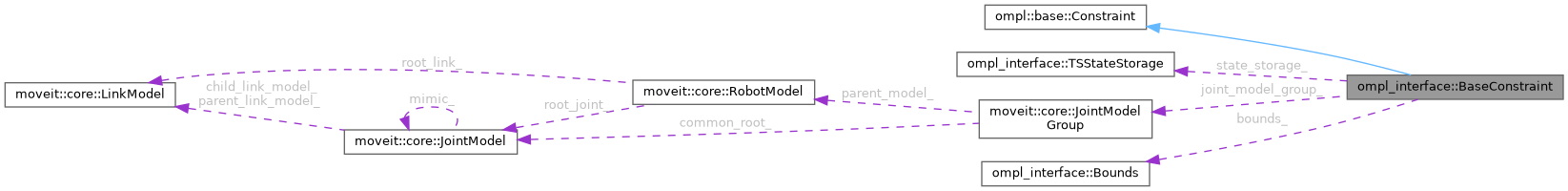[legend]

## Public Member Functions

BaseConstraint (const moveit::core::RobotModelConstPtr &robot_model, const std::string &group, const unsigned int num_dofs, const unsigned int num_cons=3)
Construct a BaseConstraint using 3 `num_cons` by default because all constraints currently implemented have 3 constraint equations. More...

void init (const moveit_msgs::msg::Constraints &constraints)
Initialize constraint based on message content. More...

void function (const Eigen::Ref< const Eigen::VectorXd > &joint_values, Eigen::Ref< Eigen::VectorXd > out) const override

void jacobian (const Eigen::Ref< const Eigen::VectorXd > &joint_values, Eigen::Ref< Eigen::MatrixXd > out) const override
Jacobian of the constraint function. More...

Eigen::Isometry3d forwardKinematics (const Eigen::Ref< const Eigen::VectorXd > &joint_values) const
Wrapper for forward kinematics calculated by MoveIt's Robot State. More...

Eigen::MatrixXd robotGeometricJacobian (const Eigen::Ref< const Eigen::VectorXd > &joint_values) const
Calculate the robot's geometric Jacobian using MoveIt's Robot State. More...

virtual void parseConstraintMsg (const moveit_msgs::msg::Constraints &constraints)=0
Parse bounds on position and orientation parameters from MoveIt's constraint message. More...

virtual Eigen::VectorXd calcError (const Eigen::Ref< const Eigen::VectorXd > &) const
For inequality constraints: calculate the value of the parameter that is being constrained by the bounds. More...

virtual Eigen::MatrixXd calcErrorJacobian (const Eigen::Ref< const Eigen::VectorXd > &) const
For inequality constraints: calculate the Jacobian for the current parameters that are being constrained.

• This error jacobian, as the name suggests, is only the jacobian of the position / orientation / ... error. It does not take into account the derivative of the penalty functions defined in the Bounds class. This correction is added in the implementation of of BaseConstraint::jacobian.
More...

const Eigen::Vector3d getTargetPosition ()

const Eigen::Quaterniond getTargetOrientation ()

## Protected Attributes

TSStateStorage state_storage_
Thread-safe storage of the robot state. More...

const moveit::core::JointModelGroupjoint_model_group_

Robot link the constraints are applied to. More...

Bounds bounds_
Upper and lower bounds on constrained variables. More...

Eigen::Vector3d target_position_
target for equality constraints, nominal value for inequality constraints. More...

Eigen::Quaterniond target_orientation_
target for equality constraints, nominal value for inequality constraints. More...

## Detailed Description

Abstract base class for different types of constraints, implementations of ompl::base::Constraint.

To create a constrained state space in OMPL, we need a model of the constraints, that can be written in the form of equality constraints F(joint_values) = 0. This class uses `Bounds` defined above, to convert:

lower_bound < scalar value < upper bound

into an equation of the form f(x) = 0.

The 'scalar value' can be the difference between the position or orientation of a link and a target position or orientation, or any other error metric that can be calculated using the `moveit::core::RobotModel` and `moveit::core::JointModelGroup`.

Definition at line 121 of file ompl_constraints.h.

## ◆ BaseConstraint()

 ompl_interface::BaseConstraint::BaseConstraint ( const moveit::core::RobotModelConstPtr & robot_model, const std::string & group, const unsigned int num_dofs, const unsigned int num_cons = `3` )

Construct a BaseConstraint using 3 `num_cons` by default because all constraints currently implemented have 3 constraint equations.

Definition at line 123 of file ompl_constraints.cpp.

## ◆ calcError()

 Eigen::VectorXd ompl_interface::BaseConstraint::calcError ( const Eigen::Ref< const Eigen::VectorXd > & ) const
virtual

For inequality constraints: calculate the value of the parameter that is being constrained by the bounds.

In this Position constraints case, it calculates the x, y and z position of the end-effector. This error is then converted in generic equality constraints in the implementation of `ompl_interface::BaseConstraint::function`.

This method can be bypassed if you want to override `ompl_interface::BaseConstraint::function directly and ignore the bounds calculation.

Reimplemented in ompl_interface::OrientationConstraint, and ompl_interface::BoxConstraint.

Definition at line 174 of file ompl_constraints.cpp.

Here is the caller graph for this function: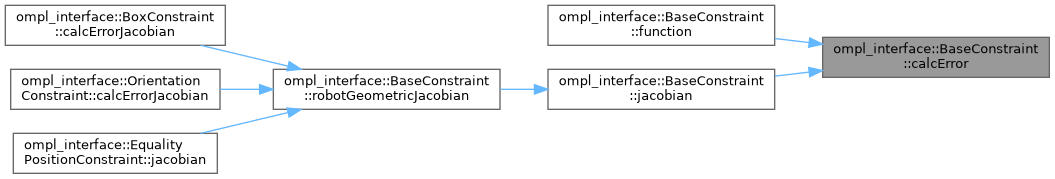## ◆ calcErrorJacobian()

 Eigen::MatrixXd ompl_interface::BaseConstraint::calcErrorJacobian ( const Eigen::Ref< const Eigen::VectorXd > & ) const
virtual

For inequality constraints: calculate the Jacobian for the current parameters that are being constrained.

• This error jacobian, as the name suggests, is only the jacobian of the position / orientation / ... error. It does not take into account the derivative of the penalty functions defined in the Bounds class. This correction is added in the implementation of of BaseConstraint::jacobian.

This method can be bypassed if you want to override `ompl_interface::BaseConstraint::jacobian directly and ignore the bounds calculation.

TODO(jeroendm), Maybe also use an output argument as in `ompl::base::Constraint::jacobian(x, out)` for better performance?

Reimplemented in ompl_interface::OrientationConstraint, and ompl_interface::BoxConstraint.

Definition at line 181 of file ompl_constraints.cpp.

Here is the caller graph for this function: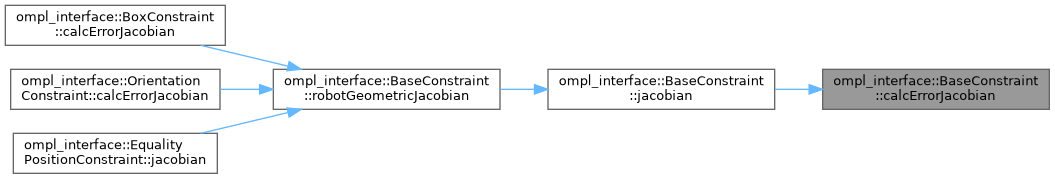## ◆ forwardKinematics()

 Eigen::Isometry3d ompl_interface::BaseConstraint::forwardKinematics ( const Eigen::Ref< const Eigen::VectorXd > & joint_values ) const

Wrapper for forward kinematics calculated by MoveIt's Robot State.

TODO(jeroendm) Are these actually const, as the robot state is modified? How come it works? Also, output arguments could be significantly more performant, but MoveIt's robot state does not support passing Eigen::Ref objects at the moment.

Definition at line 156 of file ompl_constraints.cpp.

Here is the call graph for this function: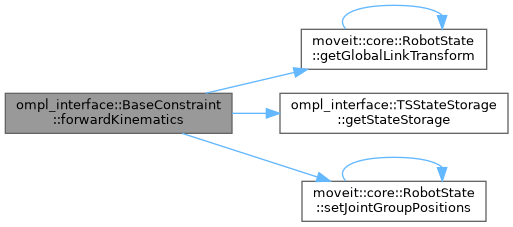Here is the caller graph for this function: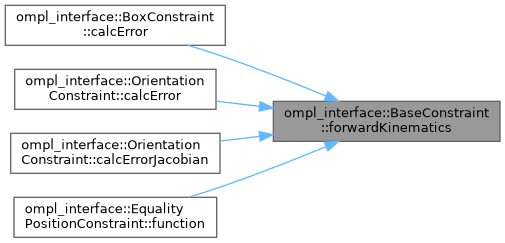## ◆ function()

 void ompl_interface::BaseConstraint::function ( const Eigen::Ref< const Eigen::VectorXd > & joint_values, Eigen::Ref< Eigen::VectorXd > out ) const
override

OMPL's main constraint evaluation function.

OMPL requires you to override at least "function" which represents the constraint F(q) = 0

Definition at line 137 of file ompl_constraints.cpp.

Here is the call graph for this function: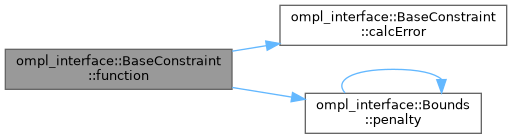inline

Definition at line 198 of file ompl_constraints.h.

## ◆ getTargetOrientation()

 const Eigen::Quaterniond ompl_interface::BaseConstraint::getTargetOrientation ( )
inline

Definition at line 208 of file ompl_constraints.h.

## ◆ getTargetPosition()

 const Eigen::Vector3d ompl_interface::BaseConstraint::getTargetPosition ( )
inline

Definition at line 203 of file ompl_constraints.h.

## ◆ init()

 void ompl_interface::BaseConstraint::init ( const moveit_msgs::msg::Constraints & constraints )

Initialize constraint based on message content.

This is necessary because we cannot call the pure virtual parseConstraintsMsg method from the constructor of this class.

Definition at line 132 of file ompl_constraints.cpp.

Here is the call graph for this function: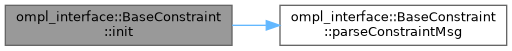## ◆ jacobian()

 void ompl_interface::BaseConstraint::jacobian ( const Eigen::Ref< const Eigen::VectorXd > & joint_values, Eigen::Ref< Eigen::MatrixXd > out ) const
override

Jacobian of the constraint function.

Optionally you can also provide dF(q)/dq, the Jacobian of the constraint.

Definition at line 144 of file ompl_constraints.cpp.

Here is the call graph for this function: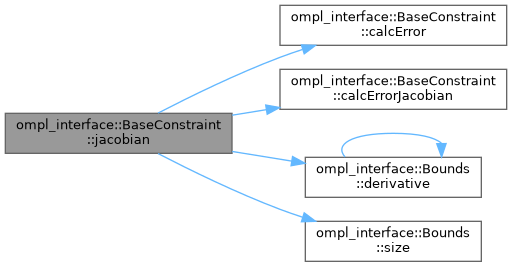Here is the caller graph for this function: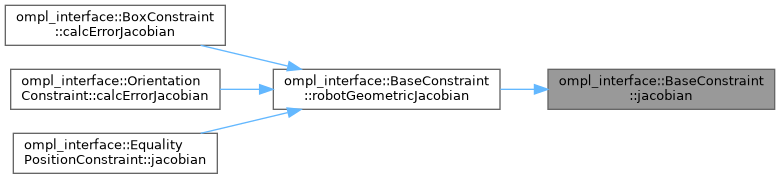## ◆ parseConstraintMsg()

 virtual void ompl_interface::BaseConstraint::parseConstraintMsg ( const moveit_msgs::msg::Constraints & constraints )
pure virtual

Parse bounds on position and orientation parameters from MoveIt's constraint message.

This can be non-trivial given the often complex structure of these messages.

Here is the caller graph for this function: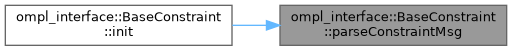## ◆ robotGeometricJacobian()

 Eigen::MatrixXd ompl_interface::BaseConstraint::robotGeometricJacobian ( const Eigen::Ref< const Eigen::VectorXd > & joint_values ) const

Calculate the robot's geometric Jacobian using MoveIt's Robot State.

Ideally I would pass the output argument from OMPL's jacobian function directly, but I cannot pass an object of type , Eigen::Ref<Eigen::MatrixXd> to MoveIt's Jacobian method.

Definition at line 163 of file ompl_constraints.cpp.

Here is the call graph for this function: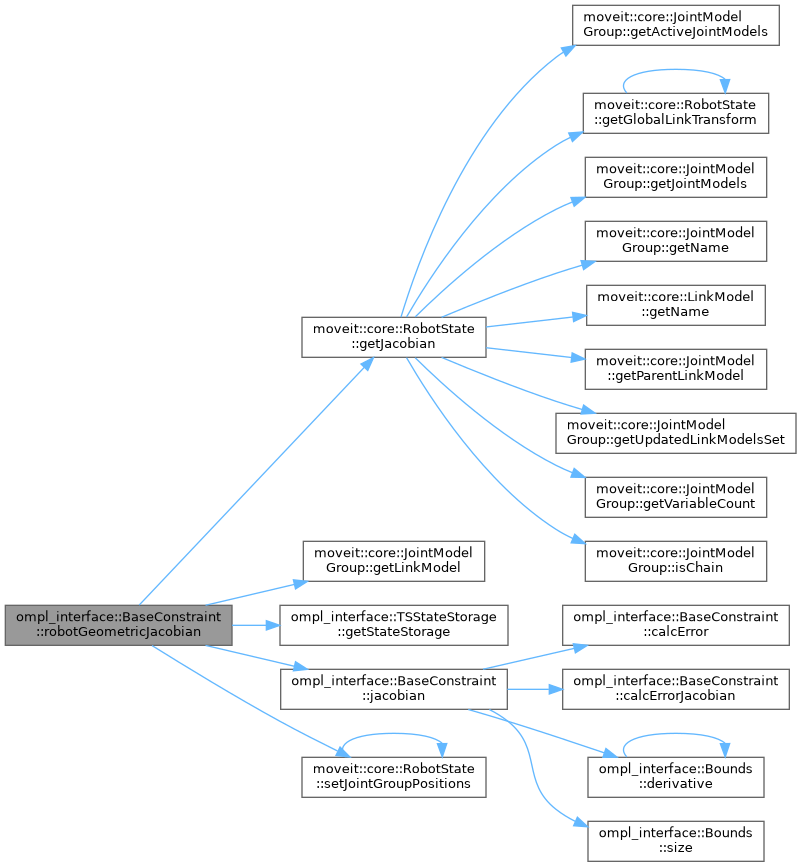Here is the caller graph for this function: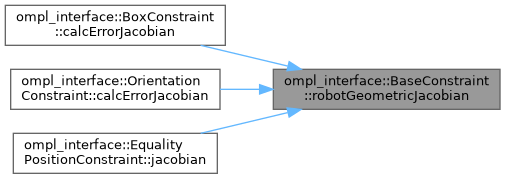## ◆ bounds_

 Bounds ompl_interface::BaseConstraint::bounds_
protected

Upper and lower bounds on constrained variables.

Definition at line 231 of file ompl_constraints.h.

## ◆ joint_model_group_

 const moveit::core::JointModelGroup* ompl_interface::BaseConstraint::joint_model_group_
protected

Definition at line 220 of file ompl_constraints.h.

protected

Robot link the constraints are applied to.

Definition at line 228 of file ompl_constraints.h.

## ◆ state_storage_

 TSStateStorage ompl_interface::BaseConstraint::state_storage_
protected

Thread-safe storage of the robot state.

The robot state is modified for kinematic calculations. As an instance of this class is possibly used in multiple threads due to OMPL's LazyGoalSampler, we need a separate robot state in every thread.

Definition at line 219 of file ompl_constraints.h.

## ◆ target_orientation_

 Eigen::Quaterniond ompl_interface::BaseConstraint::target_orientation_
protected

target for equality constraints, nominal value for inequality constraints.

Definition at line 237 of file ompl_constraints.h.

## ◆ target_position_

 Eigen::Vector3d ompl_interface::BaseConstraint::target_position_
protected

target for equality constraints, nominal value for inequality constraints.

Definition at line 234 of file ompl_constraints.h.

The documentation for this class was generated from the following files: